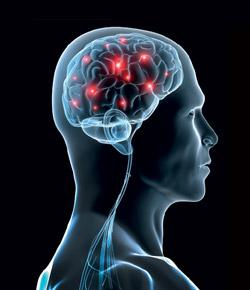# Test Your Brain With Facts And Questions About The Brain:  Neuroscience Quiz

20 Questions | Total Attempts: 743SettingsTest your brain with facts and questions about the brain !

Questions and Answers
• 1.
What if we lined up all the neurons in our body? How long would that line stretch?
• A.

100,000,000,000 neurons x 10 microns= 1000 km or about 600 miles

• B.

100,000,000,000,000 neuron x 10 microns= 5000 km or about 500 miles

• C.

10 neurons x 10 microns= 100 km or about 60 miles

• D.

1,000 neurons x 10 microns= 1000 km or about 600 miles

• 2.
What is the longest living cell in your body? This cell can live for your entire life time !
• A.

Skin Cells

• B.

Brain Cells

• C.

Blood Cells

• D.

Eye Cells

• 3.
How much does the average human brain weigh?
• A.

5 pounds

• B.

2 pounds

• C.

6 pounds

• D.

3 pounds

• 4.
What percent of the total body weight does the adult human brain account for?
• A.

3 %

• B.

5 %

• C.

12 %

• D.

2 %

• 5.
How much does the elephant brain weigh?
• A.

14 pounds

• B.

13 pounds

• C.

8 pounds

• D.

5 pounds

• 6.
How many neurons (brain cells) does an average person have ?
• A.

100 billion neurons

• B.

10 billion neurons

• C.

100 million neurons

• D.

10 million neurons

• 7.
How much area does the brain cover if laid flat?
• A.

5.5 square feet

• B.

10 square feet

• C.

2.5 square feet

• D.

5 square feet

• 8.
What's the world record for someone going without sleep?
• A.

10 days

• B.

6 days

• C.

4 days

• D.

19 days

• 9.
How many times does your eye blink in a year?
• A.

200,000 times

• B.

4,200,000 times

• C.

10,230,000 times

• D.

1,000,000 times

• 10.
How long can the brain go without blood?
• A.

4-5 seconds

• B.

5-10 minutes

• C.

8-10 seconds

• D.

1-2 minutes

• 11.
How quickly can neurons reproduce during early development?
• A.

250,000 neurons/minute

• B.

400,000 neurons/minute

• C.

1,000,000 neurons/day

• D.

53,000 neurons/day

• 12.
How much of your body heat is lost through your head?
• A.

20 - 30 %

• B.

30 - 40 %

• C.

40 - 50 %

• D.

50 - 60 %

• 13.
Does the octopus have a blind spot?
• A.

True

• B.

False

• 14.
How much does your skin alone weigh?
• A.

1 pound

• B.

2 pounds

• C.

4 pounds

• D.

6 pounds

• 15.
How many eyes to scorpions have?
• A.

2 eyes

• B.

4 eyes

• C.

8 eyes

• D.

12 eyes

• 16.
Where are the ears of cricket located?
• A.

It's head

• B.

It's front legs

• C.

It's back legs

• D.

It's brain

• 17.
What is the most complicated computer in the world?
• A.

The super computer

• B.

Your Brain

• C.

The rat's brain

• D.

The super complex computer

• 18.
How much of your calories are used up by your brain?
• A.

20 - 30 %

• B.

40 - 50 %

• C.

10 - 20 %

• D.

1 - 10%

• 19.
How many taste buds (tongue, palate, cheeks) do you have?
• A.

10,000

• B.

50,000

• C.

500,000

• D.

1,000,000

• 20.
What is the total number of smell receptor cells in your nose?
• A.

50 million

• B.

40 million

• C.

5 million

• D.

1 million

Related Topics